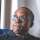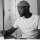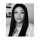## Whether or not to continue this bloody MBA programme – Episode 1

Download R, download R studio. There is no need for you to use R. R studio was made after R. it is more user friendly. We used it a long time ago before the advent of R studio. Have you opened R studio? Put in these codes (at this point, I am lost). What is going on here? Why am I hearing the word codes? Am I being played? This is a Data Analytics class right? I am supposed to be learning probability right? At the most, I will learn Statistics right? Why are you taking my whole world into coding? Can somebody get me out of here?

## The Probability of going on with the programme

Let me briefly take us through what probabilty is, then I will give an answer to whether I should continue the programme or not.

The simplest definition of probability is that it is used to quantify uncertainty. Probability works with these three things; chances, likelihood and odds. Bayesian probability is interpreted as a reasonable expectation representing a state of knowledge or as a quantification of personal belief.

## How to calculate probability

Divide the number of events by the number of possible outcomes. i.e

Probability of an event A, given that B equals the probability of Band A happening together, divided by the probability of B.

Deal with each probability separately to calculate independent events. i.e The probability of rolling (2) on a dice of (6) is calculated as 1/6.

To know the probability of rolling number (2) twice in a game should be calculated independently. 1/6 for the first and 1/6 for the other.

Dependent events; a jar contains 4 blue marbles, 5 red marbles and 11 white marbles. If 3 marbles are drawn from the jar at random, what is the probability that the first marble is red, the second is blue and the third is white?

The probability of the first marble being red is 5/20 or 1/4. The probability of the second marble being blue is 4’/19. 4 because there are only 4 blue marbles and 19 because 1 ball has been taken out when red marbles were calculated. The probability of it being a white ball is 11/18. This will be equal to 5/20*4/19*11/18 = 44/1368 = 32%.

## What is the essence of all these?

I hope I have left you as confused as I felt during this lecture. It got worse when a table was presented to us, and the faculty asked that we plot the table in a graph using excel, then import the file into R studio. R studio again?

I came here to learn how to grow and maintain my business, not how to be the one responsible to analyzing the data received from my day to day activities.

So many things ran through my mind, and just as the class ended, the thought of dropping out of the MBA programme filled my mind. There was no way I would cope with this.

The dilemma continues.## Exam Period😒 ….

in## Communicating in times of Crisis😊

inin

## 6 Replies to “Should I or Should I not?”

1.Gene says:

Okay… I’m smiling…

Nice writing.

I hope you don’t drop the bloody thing!

1.Abimbola Kayode-Badmus says:

Haha. i’m greater than the bloody thing. For now!

2.Taiwo Williams says:

Lool the dilemma continues indeed o.

3.Norah Charles says:

Bimbo…this is great. LOL! We’re doing this bloody thing till the end!

1.Abimbola Kayode-Badmus says:

I know right! Thanks Nora.

This site uses Akismet to reduce spam. Learn how your comment data is processed.